# Category theory without categories

I was bewildered by my first exposure to category theory. My first semester in graduate school I had a textbook with definitions like “A gadget is an object G such that whenever you have this unfamiliar constellation of dots and arrows, you’re allowed to draw another arrow from here to there.” What? Why?!

I revisited category theory occasionally after college, going through cycles of curiosity followed by revulsion. It took several cycles before I could put my finger on why I found category theory so foreign.

There are numerous obstacles to appreciating category theory, but the biggest may be diagrammatic reasoning. More specifically, having to learn diagrammatic reasoning at the same time as facing other challenges.

Why should diagrammatic reasoning be difficult? Isn’t the purpose of diagrams to make things clearer?

Usually diagrams are supplementary. They illustrate things that are described verbally. But in category theory, the diagrams are primary, not supplementary. Instead, you have definitions and theorems stated in the language of diagrams .

In practice, category theory uses a style of presentation you’re unlikely to have seen anywhere else. But this is not essential. You could do category theory without drawing diagrams, though nobody does. And, importantly for this post, you can use category theory-like diagrams without reference to categories. That’s what William Lawvere does in his book Conceptual Mathematics. The book uses Karate Kid-like pedagogy: the student gains fluency with a practice before being told its significance.

When you see a triangle made of two arrows, what’s the significance of the existence of an arrow that makes the diagram into a triangle? What difference does it make when the missing arrow goes on one side of the diagram or the other as in the two diagrams below?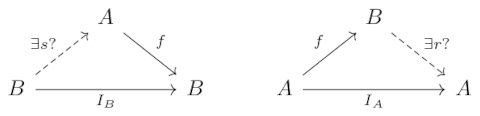The answer is not obvious if you’re unfamiliar with this way of thinking, and yet the problem has nothing to do with category theory per se. You could ask the question in the context of finite sets and functions. And that’s what Lawvere’s book does, acquainting the reader with diagrammatic reasoning before getting into category theory as such.

***

 Or more to the heart of the matter, you have definitions in terms of functions (“morphisms”) that are represented by diagrams. The difficulty does not come from the diagrams but rather from formulating things in terms of the existence and uniqueness of functions rather than more tangible arguments about sets and elements. This indirect formulation may seem unnecessary, or even sadistic, but it is generalizes further.

# Simple example of Kleisli compositionWhen a program needs to work with different systems of units, it’s best to consistently use one system for all internal calculations and convert to another system for output if necessary. Rigidly following this convention can prevent bugs, such as the one that caused the crash of the Mars Climate Orbiter.

For example, maybe you need to work in degrees and radians. It would be sensible to do all calculations in radians, because that’s what software libraries expect, and output results in degrees, because that’s what humans expect.

Now suppose you have a function that takes in a length and doubles it, and another function takes in a length and triples it. Both functions take in length in kilometers but print the result in miles.

You would like the composition of the two functions to multiply a length by six. And as before, the composition would take in a speed in kilometers and return a speed in miles.

Here’s how we could implement this badly.

    miles_per_km = 5/8 # approx

def double(length_km):
return 2*length_km*miles_per_km

def triple(length_km):
return 3*length_km*miles_per_km

length_km = 8
d = double(length_km)
print("Double: ", d)
t = triple(d)
print("Triple: ", t)


This prints

    Double: 10.0
Triple: 18.75

The second output should be 30, not 18.5. The result is wrong because we converted from kilometers to miles twice. The correct implementation would be something like the following.

    miles_per_km = 0.6213712

def double(length_km):
d = 2*length_km
print("Double: ", d*miles_per_km)
return d

def triple(length_km):
t = 3*length_km
print("Triple: ", t*miles_per_km)
return t

length_km = 8
d = double(length_km)
t = triple(d)


This prints the right result.

    Double: 10.0
Triple: 30.0


In abstract terms, we don’t want the composition of f and g to be simply gf.

We have a function f from X to Y that we think of as our core function, and a function T that translates the output. Say f doubles its input and T translates from kilometers to miles. Let f* be the function that takes X to TY, i.e. the combination of f and translation.

Now take another function g from Y to Z and define g* as the function that takes Y to TZ. We want the composition of f* and g* to be

g* ∘ f* = T ∘ g ∘ f.

In the example above, we only want to convert from kilometers to miles once. This is exactly what Kleisli composition does. (“Kleisli” rhymes with “highly.”)

Kleisli composition is conceptually simple. Once you understand what it is, you can probably think of times when it’s what you wanted but you didn’t have a name for it.

Writing code to encapsulate Kleisli composition takes some infrastructure (i.e. monads), and that’s a little complicated, but the idea of what you’re trying to achieve is not. Notice in the example above, what the functions print is not what they return; the print statements are a sort of side channel. That’s the mark of a monad.

## Kleisli categories

The things we’ve been talking about are formalized in terms of Kleisli categories. You start with a category C and define another category that has the same objects as C does but has a different notion of composition, i.e. Kleisli composition.

Given a monad T on C, the Kleisli category CT has the same objects as C. An arrow f* from X to Y in CT corresponds to an arrow f from X to TY in C. In symbols,

HomCT(X, Y) = HomC(X, TY).

Mr. Kleisli’s motivation for defining his categories was to answer a more theoretical question—whether all monads arise from adjunctions—but more practically we can think of Kleisli categories as a way of formalizing a variation on function composition.

# Getting pulled back in

“Just when I thought I was out, they pull me back in.” — Michael Corleone, The Godfather, Part 3

My interest in category theory goes in cycles. Something will spark my interest in it, and I’ll dig a little further. Then I reach my abstraction tolerance and put it back on the shelf. Then sometime later something else comes up and the cycle repeats. Each time I get a little further.

A conversation with a client this morning brought me back to the top of the cycle: category theory may be helpful in solving a concrete problem they’re working on.

I’m skeptical of applied category theory that starts with categories. I’m more bullish on applications that start from the problem domain, a discussion something like this.

“Here’s a pattern that we’re trying to codify and exploit.”

“Category theory has a name for that, and it suggests you might also have this other pattern or constraint.”

“Hmm. That sounds plausible. Let me check.”

I think of category theory as a pattern description language, a way to turn vague analogies into precise statements. Starting from category theory and looking for applications is less likely to succeed.

When I left academia the first time, I got a job as a programmer. My first assignment was to make some change to an old Fortran program, and I started asking a lot of questions about context. My manager cut me off saying “You’ll never get here from there.” I had to work bottom-up, starting from the immediate problem. That lesson has stuck with me ever since.

Sometimes you do need to start from the top and work your way down, going from abstract to concrete, but less often that I imagined early in my career.

# Galois connections and Galois theory

What are Galois connections and what do they have to do with Galois theory?

Galois connections are much more general than Galois theory, though Galois theory provided the first and most famous example of what we now call a Galois connection.

## Galois connections

Galois connections are much more approachable than Galois theory. A Galois connection is just a pair of functions between partially ordered sets that have some nice properties. Specifically, let (A, ≤) and (B, ≤) be partially ordered sets. Here “≤” denotes the partial order on each set, and so could be defined differently on A and B.

We could add subscripts to distinguish the two meanings of ≤ but this is unnecessary because the meaning is always clear from context: if we’re comparing two things from A, we’re using the ≤ operator on A, and similarly for B.

Note that “≤” could have something to do with “less than” but it need not; it represents a partial order that may or may not be helpful to think of as “less than” or something analogous to less than such as “contained in.”

### Monotone and antitone functions

Before we can define a Galois connection, we need to define monotone and antitone functions.

A monotone function is an order preserving function. That is, a function f is monotone if

xyf(x) ≤ f(y).

Similarly, an antitone function is order reversing. That is, a function f is antitone if

xy ⇔ f(x) ≥ f(y).

Here ≥ is defined by

yxxy.

### Monotone and antitone connections

Galois connections have been defined two different ways, and you may run into each in different contexts. Fortunately it’s trivial to convert between the two definitions.

The first definition says that a Galois connection between A and B is a pair of monotone functions F and G such that for all a in A and b in B,

F(a) ≤ baG(b).

The second definition says that a Galois connection between A and B is a pair of antitone functions F and G such that for all a in A and b in B,

F(a) ≤ ba ≥ G(b).

If you need to specify which definition you’re working with, you can call the former a monotone Galois connection and the latter an antitone Galois connection. We only need one of these definitions: if we reverse the definition of ≤ on B then a monotone connection becomes antitone and vice versa. 

How can we just reverse the meaning of ≤ willy-nilly? Recall that we said above that ≤ is just a notation for a partial order. There’s no need for it to mean “less than” in any sense, and the opposite of a partial order is another partial order.

We’ll use the antitone definition for the rest of the post because our examples are antitone. Importantly, the Fundamental Theorem of Galois Theory involves an antitone connection.

## Examples

For our first example, let A be sets of points in the plane, and let B be sets of lines in the plane. For both sets let ≤ mean subset.

For a set of points X, define F(X) to be the set of lines that go through all the points of X.

Similarly, for a set of lines Y, define G(Y) to be the set of points on all the lines in Y.

Then the pair (F, G) form a Galois connection.

This example can be greatly generalized. Let R be any binary relation between A and B and let ≤ mean subset.

Define

F(X) = { y | x R y for all x in X }

G(Y) = { x | x R y for all y in Y }

Then the pair (F, G) form a Galois connection. The example above is a special case of this construction where x R y is true if and only if x is a point on y. Garrett Birkhoff made this observation in 1940 .

## Galois theory

Galois theory is concerned with fields, extension fields, and automorphisms of fields that keep a subfield fixed.

I recently wrote a series of blog posts explaining what images on the covers of math books were about, and one of these posts was an explanation of the following diagram: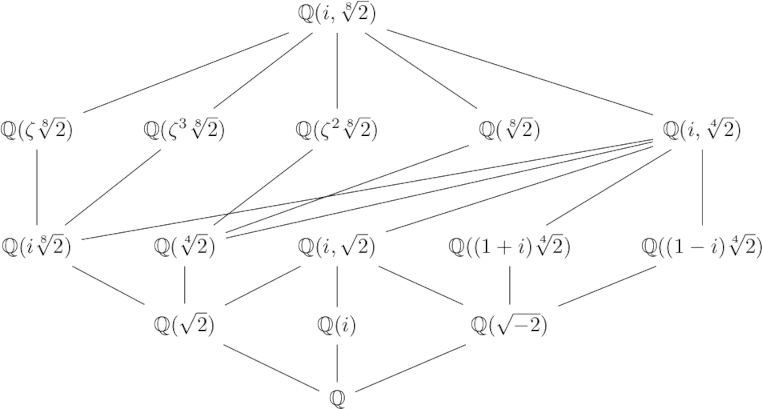Each node in the graph is a field, and a line means the field on the higher level is an extension of the field on the lower level. For each graph like this of extension fields, there is a corresponding graph of Galois groups. Specifically, let L be the field at the top of the diagram and let E be any field in the graph.

The corresponding graph of groups replaces E with the group of group isomorphisms from L to L that leave the elements of E unchanged, the automorphisms of L that fix E. This map from fields to groups is half of a Galois connection pair. The other half is the map that takes each group to the field of elements of L fixed by G. This connection is antitone because if a field F is an extension of E, then the group of automorphisms that fix F are a subgroup of the automorphisms that fix E.

***

 We could think of A and B as categories, where there is a morphism between x and y iff xy. Then a monotone Galois connection between A and B is an antitone Galois connection between A and Bop.

 Garrett Birkhoff. Lattice Theory. American Mathematical Society Colloquium Publications, volume 25. New York, 1940.

# A general theory of sub-things

When I took my first abstract algebra course, we had a homework question about subgroups. Someone in the class whined that the professor hadn’t told us yet what a subgroup was. My immediate thought was “I bet you could guess. Sub things are all the same. A sub-X is an X contained inside another X. Subset, subspace, subgroup, … They’re all the same.”

My initial reaction was right in that all sub-things are analogous. A subspace is a subset of a vector space that is a vector space in its own right, etc. But there are some hairs that are occasionally worth splitting.

If A is a sub-thing of a thing B, and a is an element of A, sometimes it’s useful to distinguish whether we’re thinking of a as an element of A or whether we’re thinking of a as an element of B. For example, the real numbers are contained in the complex numbers, and when we say -3 has no square root, we implicitly mean -3 as a real number. But if we speak of -3 in complex analysis, say as the location of a pole, we’re thinking of -3 as a complex number, a particular location in the complex plane.

This may all seem pedantic, and it may be, depending on context. But in complicated situations, it can help to make fine distinctions explicit. Novices are unaware of fine distinctions, and sophomores love to point them out, but experts know how to adjust the level of rigor to the appropriate level for a given discussion.

If we want a general theory of sub-things that spans multiple areas of math, we have to use category theory. That’s what category theory is for, codifying patterns that occur across various contexts.

But it seems we’re blocked from the beginning since objects in category theory are black boxes. You cannot speak of parts of an object, so you can’t speak of a subgroup as being a group whose elements belong to another group. You can’t speak of elements at all using the language of category theory.

The only tool at your disposal in category theory is functions. Or to be more precise, morphisms, things that act like functions but might not actually be functions.

What kind of function acts like a subset? Inclusion maps. If A is a subset of B, then there’s a function mapping A into B that sends every point to itself. Going back to the discussion above, an inclusion map is a function that takes a as a member of A to a as a member of B.

Inclusion maps are one-to-one (injective) and so maybe we could use one-to-one functions as our analog of sub-objects.

But saying a function is one-to-one requires looking inside an object and speaking of elements, and that’s not allowed in category theory. And besides, morphisms might not be functions. However, we can say what it means for a morphism to act like a one-to-one function. The categorical counterpart to an injective function is a monomorphism, sometimes just shortened to a “mono.” A subobject of a an object B is a an object A along with a monomorphism from A to B. 

By moving from injective functions to monomorphisms we’ve gained something and we’ve lost something.

We’ve gained the notion of preserving structure. For example, a subspace of a vector space isn’t just any subset of a vector space, it’s a linear subspace. In the context of linear algebra we could identify a linear subspace with an injective linear map. When morphisms are functions, they’re not arbitrary functions, but structure-preserving functions, such as linear transformations on linear spaces and continuous functions on topological spaces.

But when we move from subsets to monomorphisms we lose some specificity. If we have a monomorphism from A into B, the object A is only unique up to isomorphism. Everything in category theory is only determined up to isomorphism.

In one way this may be no loss. When we say, for example, that one group is a subgroup of another, maybe all we care about is the structure of the subgroup itself and we don’t care to distinguish isomorphic versions of the subgroup.

But if we’re talking about a manifold M being a submanifold N, we may care which submanifold it is. For example, a circle can be embedded in a torus (doughnut), and it may matter a great deal how we embed it.

If all subobjects are isomorphic, how can we tell them apart? That’s where subobject classifiers come in, a topic I may write about in the future.

## More category theory posts

 For more on subobjects, see Robert Goldblatt’s book Topoi: The Categorical Analysis of Logic.

# One diagram, two completely different meanings

I was thumbing through a new book on causal inference, The Effect by Nick Huntington-Klein, and the following diagram caught my eye.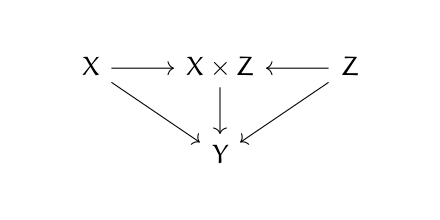Then it made my head hurt. It looks like a category theory diagram. What’s that doing in a book on causal inference? And if it is a category theory diagram, something’s wrong. Either there’s a typo or the arrows are backward.

The diagram above is a valid commutative diagram, but for a coproduct rather than a product. That is, X × Z should be labeled X ⨿ Z. (For more on that, see my post on categorical products, and reverse all the arrows in your mind.)

But there’s no category theory going on here. This is an influence diagram. It says that X and Z influence Y directly (indicated by the diagonal arrows), but they also determine the product X × Z (the ordinary product of two numbers, no fancy category stuff) and this product in turn also influences Y.

# Four, five, and nine lemmas

This post is similar in spirit to the previous post: reducing mathematical theorems to moves in a board game by looking at things from a ridiculously high level.

The theorems we’ll be looking at are known as the four lemma, the five lemma, and the nine lemma. The nine lemma is also known as the 3×3 lemma.

All the lemmas start with a commutative diagram. A diagram is commutative if any two ways of getting from one place to another are equal. If the arrows represent functions, then the diagram implies certain compositions of functions are equal.

Given hypotheses about most of the arrows in a diagram, the theorems conclude something about the rest of the arrows. This general category of theorems is known as diagram chasing. The proofs are tedious but shallow. If a theorem in topology, for example, were formulated as a diagram chase, someone with no intuition for topology could prove the theorem by applying the rules of a simple game.

The meaning of the dots and arrows isn’t important for this post, but you could think of the dots in the diagram as groups and the arrows as homomorphisms between groups. More generally you could think of the dots as objects in an Abelian category and the arrows as morphisms between objects.

## The four lemma

The four lemma starts with a commutative diagram of the following shape.It’s called the four lemma because there are four dots across each row.

There are two versions of the four lemma. Both make assumptions about the rows and three out of the four columns and conclude something about the fourth column.In the first part of the lemma, we have hypotheses about the two rows, and hypotheses about the first, third, and fourth vertical arrows. The conclusion is something about the second vertical arrow.

The second part of the lemma is very similar, but has hypotheses about the first, second, and fourth vertical arrows and concludes something about the third arrow.## Five lemma

The five lemma starts with a commutative diagram with five objects across the top and bottom rows.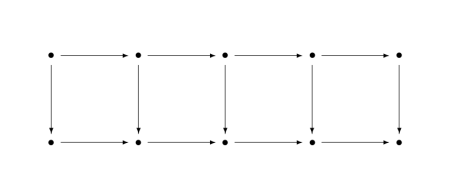Given assumptions about every arrow except the vertical arrow in the middle, the theorem concludes something about the middle arrow.## Nine lemma

The nine lemma, a.k.a. the 3×3 lemma, starts with a commutative diagram of the following shape.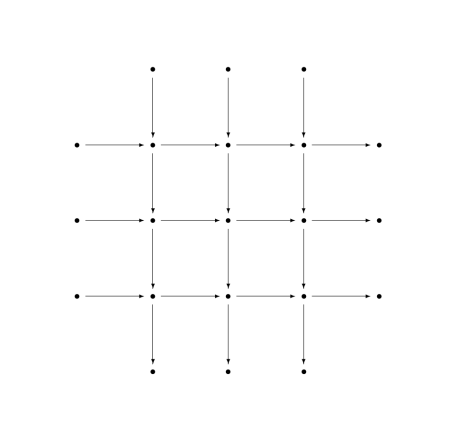It’s called the nine lemma because the attention is focused on the nine dots, the 3×3 grid of dots in the middle. All the objects around the perimeter, those outside the 3×3 grid, are 0.

There are three parts to the nine lemma. Each has hypotheses about all the columns and two of the rows, and concludes something about the row that was left out. So, for example, one part of the theorem has hypotheses about the three columns and the first two rows and concludes something about the last row.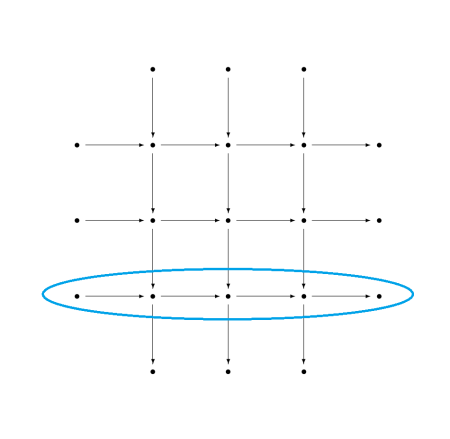## Missing details

Here I zoom in from 100,000 feet to 10,000 feet, giving some of the missing details but not all. For full details, you could look up the theorems on Wikipedia or on nLab.

As promised at the beginning, this was a ridiculously high-level view of these theorems. The purpose was to point out the core of each theorem and making it possible to compare the three theorems at the highest level.

When I said a theorem makes a hypothesis about a row or a column, the hypothesis is that the row forms an exact sequence. The conclusions in the nine lemma are that a row is an exact sequence.

When I alluded to hypotheses about vertical arrows, these hypotheses are essentially saying that a function is one-to-one (injective), onto (surjective), or one-to-one and onto (bijective). More precisely, they are assumptions that a morphism is mono, epi, or iso. More on this terminology here. The conclusion of the four lemma is that an arrow is either epi or mono. The conclusion of the five lemma is that an arrow is iso.

## Related posts

In my previous post, I looked at the map Δ that takes a column vector to a diagonal matrix. I even drew a commutative diagram, which foreshadows a little category theory.

Suppose you have a function f of a real or complex variable. To an R programmer, if x is a vector, it’s obvious that f(x) means to apply f to every component of a vector. Python (NumPy) works the same way, and calls this broadcasting. To a mathematician, this looks odd. What does the logarithm of a vector, for example, even mean?

As in the previous post, we can use Δ to formalize things. We said that Δ has some nice properties, and in fact we will show it is a functor.

To have a functor, we have to have categories. (Historically, functors came first; categories were defined in order to define functors.) We will define C to be the category of column vectors and M the category of square matrices as before. Or rather, we should say the objects of C are column vectors and the objects of M are square matrices.

Categories need morphisms, functions between objects . We define the morphisms on C to be analytic functions applied componentwise. So, for example, if

z = [1, 2, -3],

then

tan(z) = [tan(1), tan(2), tan(-3)].

The morphisms on M will be analytic functions on square matrices, not applied componentwise but applied by power series. That is, given an analytic function f, we define f of a square matrix X as the result of sticking the matrix X into the power series for f. For an example, see What is the cosine of a matrix?

We said that Δ is a functor. It takes column vectors and turns them into square matrices by putting their contents along the diagonal of a matrix. We gave the example in the previous post that [4, i, π] would be mapped to the matrix with these elements on the diagonal, i.e.That says what Δ does on objects, but what does it do on morphisms? It takes an analytic function that was applied componentwise to column vectors, and turns it into a function that is applied via its power series to square matrices. That is, starting with a functionwe define the morphism f on C byand the morphism Δ f on M bywhere Z is a square matrix.

We can apply f to a column vector, and then apply Δ to turn the resulting vector into a diagonal matrix, or we could apply Δ to turn the vector into a diagonal matrix first, and then apply f (technically,  Δf). That is, the follow diagram commutes:## Python example

Applying an analytic function to a diagonal matrix gives the same result as simply applying the function to the elements of the diagonal. But for more general square matrices, this is not the case. We will illustrate this with some Python code.

    import numpy as np
from scipy.linalg import funm

d = np.array([1, 2])
D = np.diag(d)
M = np.array([[1, np.pi], [2, 0]])


Now let’s look at some output.

    >>> np.sin(d)
array([0.84147098, 0.90929743])

>>> np.sin(D)
array([[0.84147098, 0.        ],
[0.        , 0.90929743]])

>>> funm(D, np.sin)
array([[0.84147098, 0.        ],
[0.        , 0.90929743]])


So if we take the sine of d and turn the result into a matrix, we get the same thing as if we turn d into a matrix D and then take the sine of D, either componentwise or as an analytic function (with funm, function of a matrix).

Now let’s look at a general, non-diagonal matrix.

    >>> np.sin(M)
array([[0.84147099, 0],
[0.90929743, 0]])

>>> funm(D, np.sin)
array([[0.84147098, 0.        ],
[0.        , 0.90929743]])


Note that the elements in the bottom row are in opposite positions in the two examples.

 OK, morphisms are not necessarily functions, but in practice they usually are.

# Drawing commutative diagrams with Quiver

I recently discovered quiver, a tool for drawing commutative diagrams. It looks like a nice tool for drawing diagrams more generally, but it’s designed particularly to include the features you need when drawing the kinds of diagrams that are ubiquitous in category theory.

You can draw diagrams using the online app and export the result to LaTeX (tikz).

Here are some examples.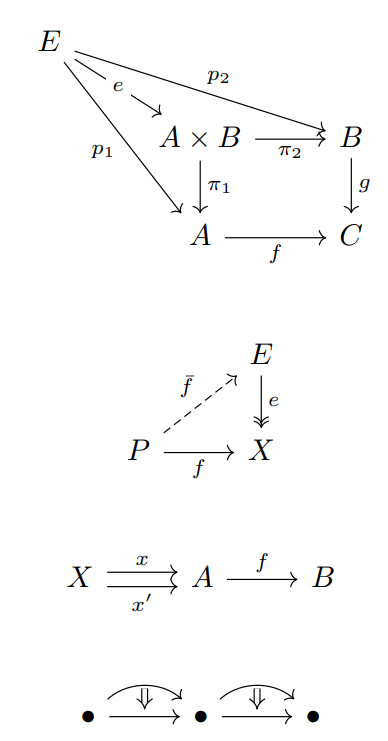The app is mostly easy to figure out. The object and arrow labels are specified using LaTeX.

Quiver lets you position labels on either side of an arrow, or even inside the arrow as in the e in the first diagram above.

You can change the arrow and arrowhead styles, as in the second example.

It took me a little while to figure out how to have two arrows with the same destination and source, as in the third example. You use the “offset” setting to move arrows up or down do that they’re not on top of each other. And by default, the software helpfully assumes that if you want to move one arrow up a bit from center, you probably want to move the other one down from center by the same amount.

You can use the “curve” setting to draw arrows as in the final example. You can also draw arrows between arrows, as is common when working with 2-categories.